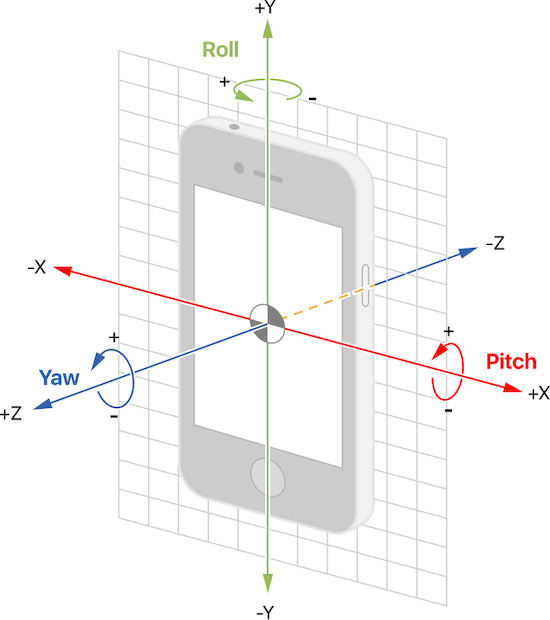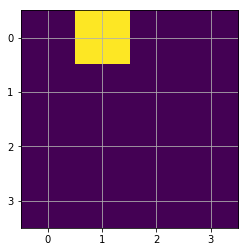# Real-Time Motion Correction— Pixel Shift — iOS

The objective is to implement a motion correction algorithm for lateral movements, in real-time, on an iOS device. The algorithm in this post can be tested by downloading MCorr, an iOS app. Lateral movements and rotational movements around the x, y and z axes are shown in Figure 1. with respect to the camera phone.

Pixel shift correction is a software based motion correction algorithm that approximates x and y axis lateral device motion. Camera motion can be corrected for by shifting the frame an optimal number of pixel, in the x or y direction, to maintain a stable video feed. This can be useful for taking videos at high zoom levels or to correct for “shaky” hands.Figure 1. rotation around the x, y, and z axes (or pitch, roll, and yaw)

The pixel shift values are with respect to the previous frame. For example, moving the camera to the left should result in positive y shifts, and moving the camera back to the original position should have negative y shifts. Please note that horizontal motion corresponds with a y shift because it shifts the columns of the image.

Relatively slow movements can produce many small, un-noticable, corrections because the camera phone captures many frames per second. One potential fix is to scale the pixel shifts as follows: (pixel shift)*(# frames)/(# seconds + 1). This makes the pixel shift values proportional to the number of frames, but can produce a large, noisy pixel shift correction. The pixel shifts were smoothed using exponential average between the current and previous shift corrections. A 50% weight was assigned to the current frames pixel shift correction in the exponential average.

The real-time motion correction was achieved by using an exponential average between the current and previous shift corrections. A 90% weight was assigned to the current frames pixel shift correction in the exponential average. Motion correction relative to a reference frame was achieved by accumulating pixel shifts. For example, moving the camera to the left should accumulate positive y shifts and moving the camera back to the original position should decrease the y shifts back to 0. Please note that horizontal motion corresponds with a y shift because it shifts the columns of the image.

# Calculating the Pixel Shift from Phase Correlation

The maximum normalized correlation between two arrays, i.e., A and B, can be found by converting the matrices into the Fourier domain. The two matrices can be converted into the Fourier domain using a Fast Fourier transform, or FFT. Correlation between these matrices can be computed in this domain by multiplying matrix A and the conjugation of matrix B. The phase correlation can be found by dividing this result by the absolute magnitude of the Fourier domain correlation matrix. Finally, the matrix is converted back to the regular domain with an inverse Fast Fourier transform, IFFT. Finally, a FFT shift operation is applied to the result. The location of maximum phase correlation in the pixel domain can be used to infer the optimal pixel shift between the two matrices.

`a:[[0 0 0 0] [0 1 0 0] [0 0 0 0] [0 0 0 0]]b:[[0 0 0 0] [0 0 0 0] [0 0 0 0] [0 0 1 0]]phase shift of `a` relative to `b`x shift: 2.0 y shift: 1.0`

The optimal pixel between matrix A relative to B is shown in the code block above. The pixel shift was found when matrix A is shifted down 2 pixels and shift to the right 1 pixel. If matrix B is the current frame, of the video feed, and matrix A is the previous frame, the motion corrected image can be display by applying the pixel shift to matrix B.

# Algorithm Details

A FFT shift operation is performed on after the phase correlation matrix is converted into the pixel domain with a IFFT. The FFT shift algorithm is used to adjust the phase correlation matrix so that the pixel shift can be interpreted with respect to the center of the image. The optimal pixel shift is found by subtracting the center coordinate of the image by the location of the maximum phase correlation.Phase correlation matrix before (left) and after (right) the FFT shift operation

The FFT shift algorithm divides the phase correlation matrix into four quadrants: 0, 1, 2, 3. The quadrants are listed in clockwise order and quadrant 0 is the upper left quadrant. It then swaps the values of the following quadrant pairs: (0,3) (1,2). The image above (left) shows the phase correlation matrix, in the pixel domain, before the FFT shift algorithm. The maximum value is located at point (2,3) in quadrant 3. The image above (right) shows the phase correlation matrix after the FFT operation. Notice how quadrant 3 swapped with quadrant 1 and the maximum value change to point (0,1).

The video at the beginning of the article uses OpenCV to perform phase correlation and to find the optimal pixel shift. The library efficiently computes the phase correlation using C++. OpenCV’s implementation preprocesses the image with zeros before applying the phase correlation algorithm. It also uses 5x5 moving average window to improve the location estimate of the peak phase correlation.